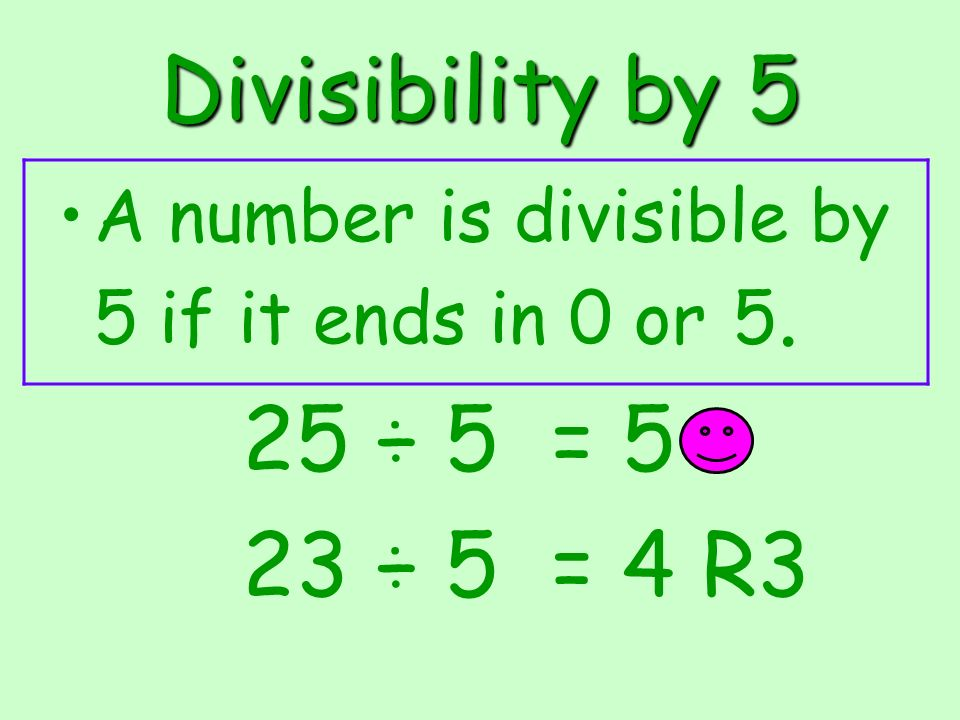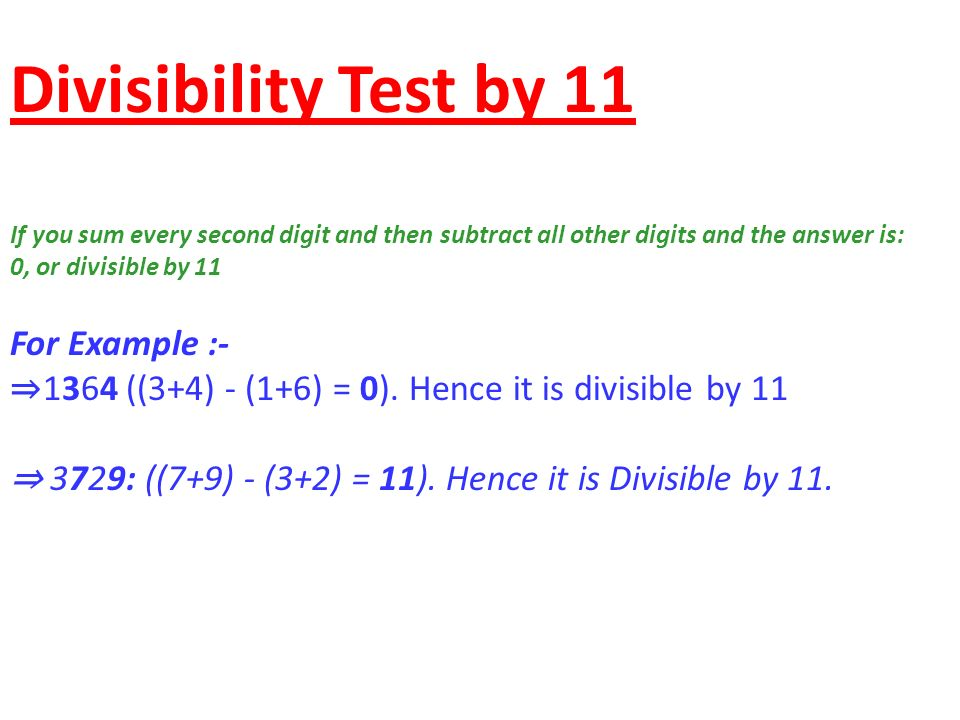# C Program To Check Number Is Divisible By 5 and 11 or Not | C Programs

C program to check whether a number is divisible by 5 and 11 or not – In this article, we will detail in on the only way to determine whether a number is divisible by 5 and 11 or not.

Suitable examples and sample programs have also been added so that you can understand the whole thing very clearly. The compiler has also been added with which you can execute it yourself.As you can see, these photos describe how a number is divisible by 5 and 11 respectively.

Divisible by 5: A number is divisible by 5 if its Unit digit is 5 or 0. For e.g. 20, 55, 75 etc.

Divisible by 11: A number is divisible by 11 if the difference between the sum of its even digits and odd digits is 0 or a multiple of 11.

The combination of these would make the number divisible by 5 and 11 both at the same time.

Thus, the only way to find out whether a number is divisible by 5 and 11 in C programming here is as follows:

## Using Standard Method

1)Read entered number n using “scanf” function.

2)The remainder of n/5 and remainder of n/11 is 0 then print “it is divisible by 5 and 11”.otherwise print “it is not divisible by 5 and 11”.

Output:
x

## C Program To Print Number Of Days In A Month | Java Tutoring

C program to input the month number and print the number of days in that ...